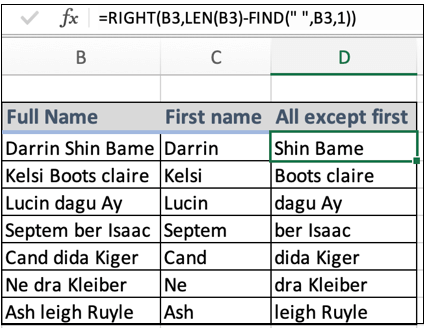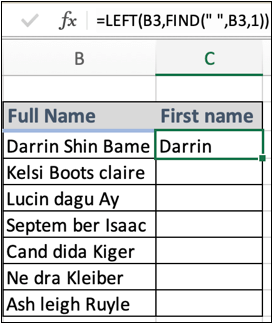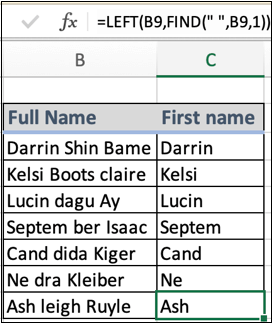# How to Extract all the words except the first in ExcelIn this article, we will learn How to Extract all the words except the first in Excel.

Scenario :

Working with text data in excel. Sometimes we come across  the problem of splitting words from the full text. For example splitting full names into first name and last name. Or extracting the names from the given email ids. For situations like these we use LEFT and RIGHT functions in excel along with some basic sense of how excel functions work. How to recognize where to separate the full name like Nedra Kleiber. Here a space character in between the first name and last name. So we direct excel to find the position of first space char in the full name and separate it into two using functions

First name and rest name formula in Excel

Here given a name value as text in the formula. First of all the find function gives the position of first space character and LEN function gives the length of full text. Now the RIGHT function has everything to extract the word.

Remaining name formula syntax:

 =RIGHT (text, LEN (text) - FIND (" ", text, 1 ) )

text : full name

" " : Space character given in quotes. Or use char function

1 : nth occurrence of space char. (here 1 is used to specify first occurrence)

First name formula syntax:

 =LEFT (text, FIND (" ", text, 1 ) )

text : full name

" " : Space character given in quotes. Or use char function

1 : nth occurrence of space char. (here 1 is used to specify first occurrence)

Example :

All of these might be confusing to understand. Let's understand how to use the function using an example. Here we are given some sample names and we need to extract the first name and rest of the name in another column. So first we start with a basic formula that is first name extraction. You can provide text values using text in quotes or by using cell reference which is explained here.

First name formula:

 =LEFT (B3, FIND (" ", B3, 1 ) )Copy and paste the formula in other cells either using CTRL + D or just by stretching the right bottom box of the C3 cell.As you can see in the above snapshot all the first names are here. Now either you can substitute the first name from the full name with blank to get the last name or use the last name formula explained below.

To get the Last name directly from the full name using the RIGHT function formula is shown below.

Remaining or last name formula:

 =RIGHT (B3, LEN (B3) - FIND (" ", B3, 1 ) )

Copy and paste the formula to different required cells using Ctrl + D or dragging down the right bottom corner of the D3 cell.As you can see the first name and last name both in different columns separated using the simple excel formulas. Learn more about excel formulas here.

Here are all the observational notes using the formula in Excel
Notes :

1. Text values in formula be used with between quotes or using cell reference. Using cell reference in excel is the best common practice.
2. Use the =CHAR(32) function to get the space character in the formula.

Hope this article about How to Extract all the words except the first in Excel is explanatory. Find more articles on calculating values and related Excel formulas here. If you liked our blogs, share it with your friends on Facebook. And also you can follow us on Twitter and Facebook. We would love to hear from you, do let us know how we can improve, complement or innovate our work and make it better for you. Write to us at info@exceltip.com.

Related Articles :

Data Validation in Excel : Data Validation is a tool used to restrict users to input value manually in cell or worksheet in Excel. It has a list of options to choose from.

Way to use Vlookup function in Data Validation : Restrict users to allow values from the lookup table using Data validation formula box in Excel. Formula box in data validation allows you to choose the type of restriction required.

Restrict Dates using Data Validation : Restrict user to allow dates from a given range in cell which lays within Excel date format in Excel.

How to give the error messages in Data Validation : Restrict users to customize input information in the worksheet and guide the input information through error messages under data validation in Excel.

Create Drop Down Lists in Excel using Data Validation : Restrict users to allow values from the drop down list using Data validation List option in Excel. List box in data validation allows you to choose the type of restriction required.

Popular Articles :